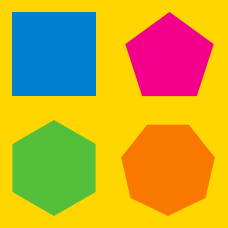Geometry

Regular Polygons: Level 3 Challenges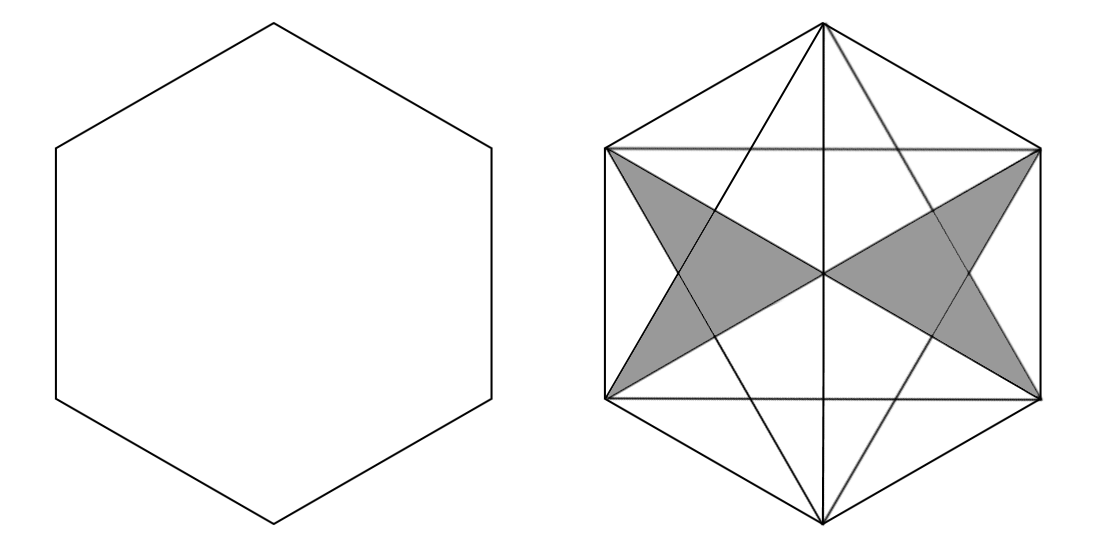The diagram above shows a regular hexagon ${ H }_{3 }$ with area $H$ which has six right triangles inscribed in it. Let the area of the shaded region be $S$, then what is the ratio $H:S?$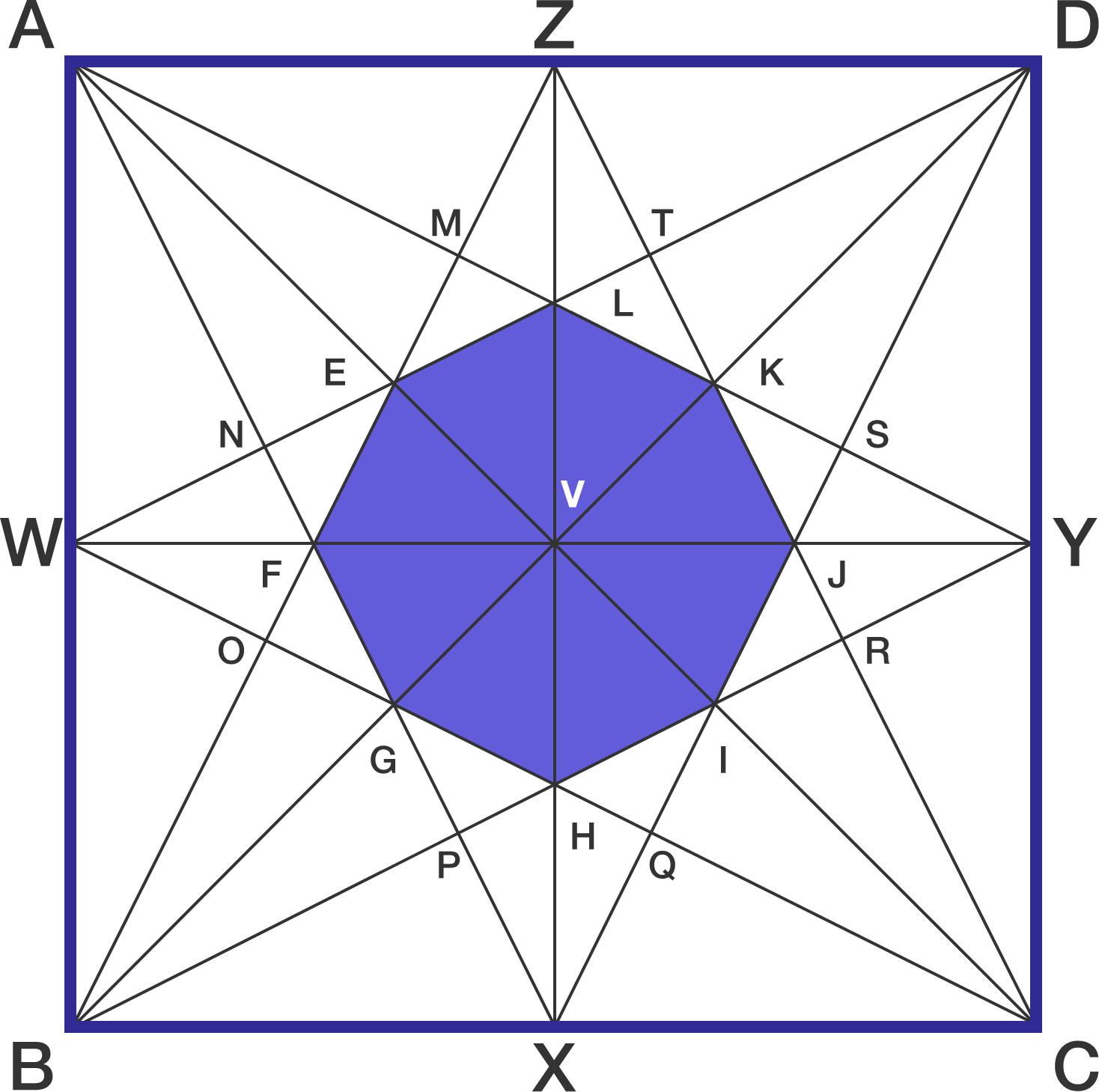Above figure shows a unit square $ABCD$.

If the area of the octagon $EFGHIJKL$ (in blue) can be expressed as $\dfrac{1}{a}$ , find $a$.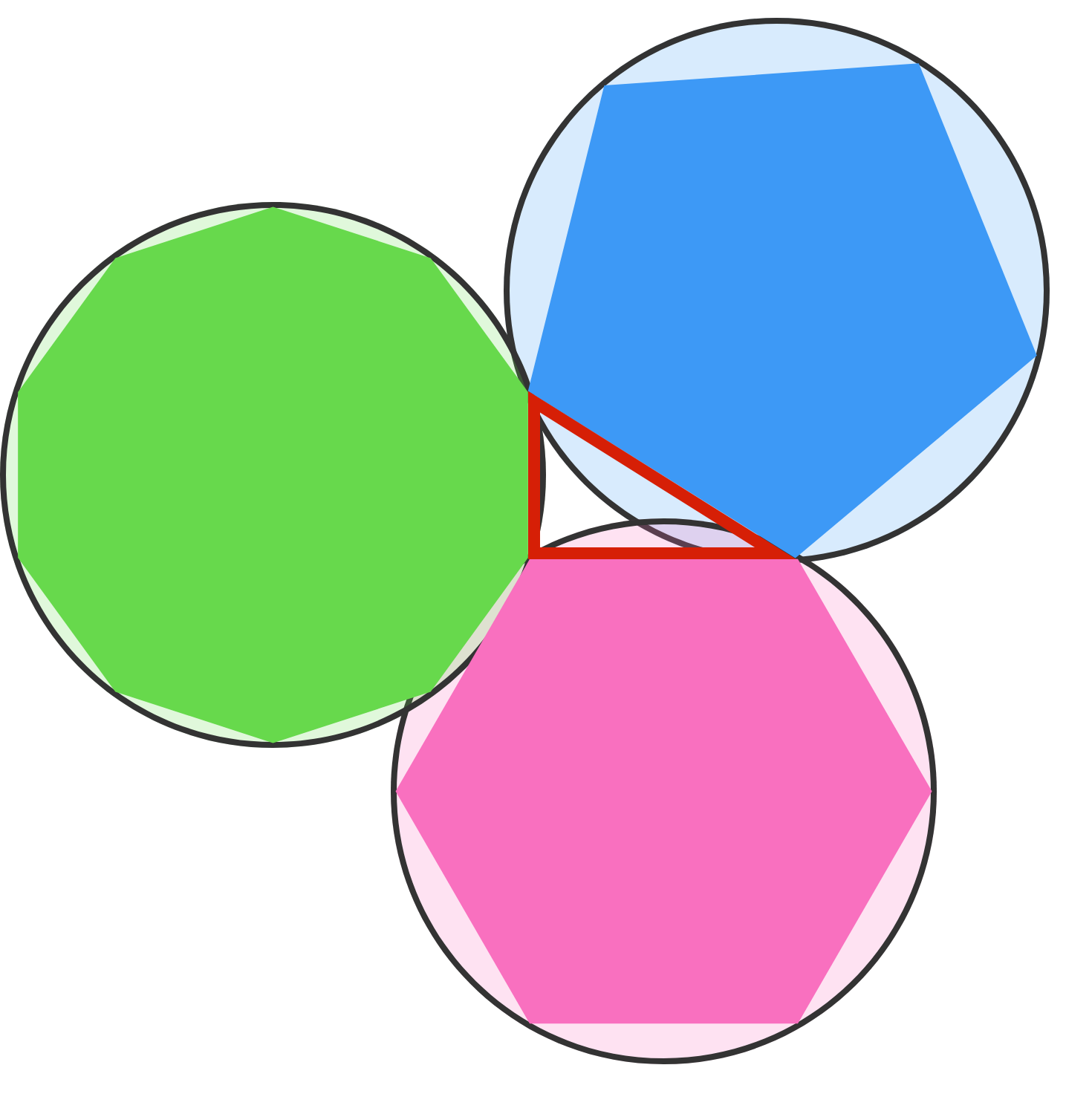As shown in the image above, a pentagon, hexagon and decagon are inscribed in three congruent circles, and their endpoints are connected to form a triangle. If the radii of each of the circles is $1$ and the area of the triangle formed by the three polygons can be written as $\frac{\sqrt{a}-b}{c}$, where $a$, $b$ and $c$ are coprime integers, what is $a+b+c$?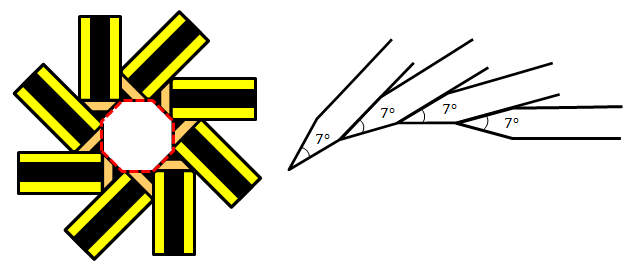We can make "pencilogons" by aligning multiple, identical pencils end-of-tip to start-of-tip together without leaving any gaps, as shown above, so that the enclosed area forms a regular polygon (the example above left is an 8-pencilogon).

Hazri wants to make an $n$-pencilogon using $n$ identical pencils with pencil tips of angle $7^\circ.$ After he aligns $n-18$ pencils, he finds out the gap between the two ends is too small to fit in another pencil.

So, in order to complete the pencilogon, he has to sharpen all the $n$ pencils so that the angle of all the pencil tips becomes $(7-m)^\circ$.

Find the value of $m+n$.

(Assume the pencils have a rectangular body and have their tips resembling isosceles triangles)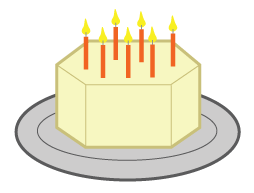Greedy Garisht is celebrating his birthday with 6 of his friends. His mother baked him a birthday cake in the shape of a regular hexagon. Wanting to keep most of the cake, he makes cuts linking the midpoints of every 2 adjacent sides, and distributes these 6 slices to his friends. What proportion of the cake does he have left for himself?

×